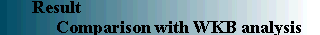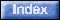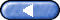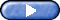In the case where the mean flow oscillation appeared, the propagation of gravity waves and the change in the mean flow were calculated with the WKB analysis, to compare them with the experimental results. In the WKB analysis, it was assumed that both the rightward-propagating wave and the leftward-propagating wave have equal amplitudes. And the deformation of the wave propagating through the mean flow, whose distribution is obtained experimentally, is calculated with the WKB analysis. Furthermore, the convergence and divergence of the momentum flux and the pattern of the mean flow acceleration were calculated for comparison with the deformation rate of the mean flow obtained experimentally. The results are shown in Figure 9-1 through Figure 9-5. Both the pattern of the wave and the convergence and divergence of the momentum flux were found to agree fairly well. There is a large discrepancy in the convergence and divergence of the momentum flux near the vibration membrane (the wave source), but it is assumed that this is caused by the large error in the velocity measurement of the fluid due to difficulty in measuring the velocity in this area.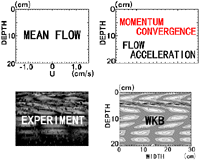Figure 9 Comparison between the figure by the moíre method and WKB analysis